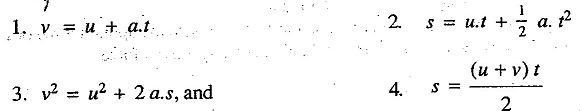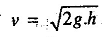### Equations of Linear Motion

What are equations of linear motion? How can we use these equations to find displacement, velocity, and acceleration?

Following are the equations of linear motion:where
u = Initial velocity,
v = Final velocity,
a .= Acceleration, and
s = Displacement of the body in time t seconds:

Notes:
1. The above equations apply for uniform acceleration.
2. In case of Vertical motion, the body is subjected to gravity. Thus the acceleration due to gravity (g) should be substituted in place of a, in the above equations.
3. The value of g is taken as + 9.81 m /2 for downward motion, and -9.81 m/ s2 for upward motion.
4. When a body falls from a height h its velocity v with which it will hit the ground, is given by